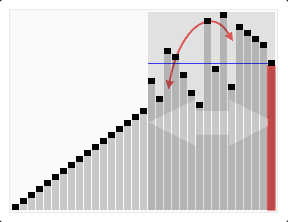There was a quicksort question posted to the LFE mail list this morning, and since I had a little time and have been wanting to try my hand at LFE more lately, I decided to answer. Upon request, this has been converted into a post for the LFE blog.

The original question was that a new LFE user had implemented a Quicksort algorithm, but had run into an issue where it seemed like the code ran in an infinite loop. Here is the code that was shared:

``````(defun partition
([_ '() small large]
(tuple small large))
([piv lst small large]
(cond
((=< (car lst) piv)
(partition piv (cdr lst) (cons (car lst) small) large))
((> (car lst) piv)
(partition piv (cdr lst) small (cons (car lst) large))))))

; Compiles but maybe enters in an infinite loop??
(defun qs
(['()] '())
([lst]
(let (((tuple small large) (partition (car lst) lst '() '())))
(++  (qs small) (car lst)  (qs large)))))``````

The problem is due to the fact that partition does not always end up generating an empty list. Take the following case:

``(partition '(3))  =>  #(() (3))``

This means you'll keep looping forever trying to partition the `'(3)` list (which will generate the same result over and over) and will never succeed.

There're two ways to fix it. One is to add a one-element list matching clause:

``````(defun qs
(['()] '())
([`(,x)] `(,x))
([list] ...)``````

Which will capture that pesky `'(3)` and cut its life short.

The other one is instead to consume the elements as you go. Now you're almost there in your code, but the problem is that while you compare `lst` to `(car lst)`, you never properly head towards generating empty lists. If you compared `(car lst)` to `(cdr lst)` and appended the head yourself, it would work.

Here's such a rewrite:

`````` (defun qs
(['()] '())
([`(,h . ,t)]
(let ((`#(,smaller ,larger) (partition h t '() '())))
(++  (qs smaller) (list h) (qs larger)))))``````

See the difference? I use `h` as a pivot, but keep it out of the partitioning business. This ensures that this:

``(partition '(3))  =>  #(() (3))``

eventually leads to having this get run:

``'(++ () (3) ())``

And it terminates.

The classic Erlang example also shows it as a nifty pair of list comprehensions, which is slower but terser, by inlining partitioning in two lists traversals being used as a filter:

``````(defun qs
(['()] '())
([`(,h . ,t)]
(++ (qs (list-comp [(<- x t) (< x h)] x))
(list h)
(qs (list-comp [(<- x t) (>= x h)] x)))))``````

But to save typing, using Erlang's lists:partition function would have been good too:

``````(defun qs
(['()] '())
([`(,h . ,t)]
(let ([`#(,smaller ,larger)
(lists:partition (lambda (x) (< x h)) t)])
(++ (qs smaller) (list h) (qs larger)))))``````

A good follow-up exercise is to create your own version of `lists:partition/2`, which accepts any one-argument predicate and splits the list in two according to this criteria.

Enjoy!

18 July 2015

tutorials